=== Introduction ===

Modern day secondary storage drives, like SSDs, divide up their storage space into fixed-sized sectors. On floppy disks and old hard disks, each sector was 512 bytes (these days they're 4KB each). When the operating system wants to access the drive, it needs to specify the sector it wants to access. Sectors are numbered from 0 up to X-1, where X is the total number of sectors on the drive. The number of a sector is called its logical block address, or LBA for short. The total storage capacity of a drive is calculated as X * sector_size.

However, it wasn't always this way. Sectors used to be addressed using three numbers, which for now we'll call C, H and S. In this blog post, I'm going to try to explain how this works, and why I hate it. This post will only cover CHS on IBM PCs; it might have worked differently on your favourite system of yore.

=== Converting between CHS and LBA ===

The conversion from CHS to LBA depends on two properties of the "geometry" of the drive, called HPC and SPT. The conversion itself is a fairly simply affair.

To convert from CHS to LBA, we use the formula:

LBA = (C * SPT * HPC) + (H * SPT) + (S - 1)

To convert from LBA to CHS, we use the formulae:

C = (LBA / SPT) / HPC
H = (LBA / SPT) % HPC
S = (LBA % SPT) + 1

We can think of C-H-S as 3 digits, with S ranging from 1 up to SPT, H ranging from 0 up to HPC - 1, and C starting at 0 and going up to whatever is necessary to index all sectors on the drive. As such, it is a fairly standard modular arithmetic affair, with the only complication being that S inexplicably starts at 1.

Why do we want to convert between CHS and LBA? Well, CHS would be a completely bonkers way for the operating system to keep track of where files are located on a drive. Not only would it massively complicate writing file system drivers, it would also have the benefit of making a file system dependent on the drive on which it is created (because it relies on the drive's geometry).

=== HPC and SPT ===

When you ask the BIOS for the geometry of a drive, instead of giving you the values of HPC and SPT, it unhelpfully gives the maximum value H and S can respectively take. The value of HPC is one more than the maximum value of H (since H goes from 0 to HPC - 1). The value of SPT however is the same as the maximum value of S, because S starts at 1.

=== Eating the fruit of the tree whereof I said thou shouldst not eat ===

If you haven't lost all hope in technology yet, don't worry. It's about to get a lot worse. I must now uncover the terrible reality which I have kept hidden from you. I will now explain what CHS, HPC and SPT stand for.

C: Cylinder
S: Sector
SPT: Sectors per track

Oh dear.

=== A floppy disk ===

A floppy disk contains a thin circular disk of a magnetic material. Data is read and written by a read head, part of the floppy drive (please ignore that the "read" head does both reading and writing). The data on the disk is divided in 512 byte sectors. To make efficient use of the space, sectors are placed on both sides of the disk. On a given side, each sector belongs to a track, a group of sectors that all the same distance from the centre of the disk.As you may see in the above diagram, the number of sectors in each track is kept constant. This means that the sectors on the tracks close to the center of the disk are physically smaller. There is some empty space left in the centre, because if sectors were placed there, the timing of accessing them would be too precise.

Thus, to uniquely identify a sector on a floppy disk, we need precisely 3 pieces of information:
1. Which side of the disk the sector is on.
2. How far the sector is from the center of the disk (radius).
3. The anticlockwise angle, starting from the positive x axis, which we must go to reach the sector.

These are in fact the values we'll use for CHS addressing! (1) corresponds to H, the "head"; (2) corresponds to C, the "cylinder"; and (3) corresponds to S, the "sector" (very aggressive air quotes for this one).

=== Cylindrical coordinates ===

Sectors are arranged on a hard disk in a very similar fashion to a floppy disk. The main difference is that in a hard disk there are multiple magnetic disks stacked on top of each other. So we can extend our above addressing format to deal with hard disks by changing (1) to give the depth of the sector from the top of the stack.

With this, we are identifying each sector with a depth-radius-angle triplet. In mathematics, this is a fairly standard way to identify points on a cylinder, which is a good way to visualize the arrangement of sectors in a hard disk.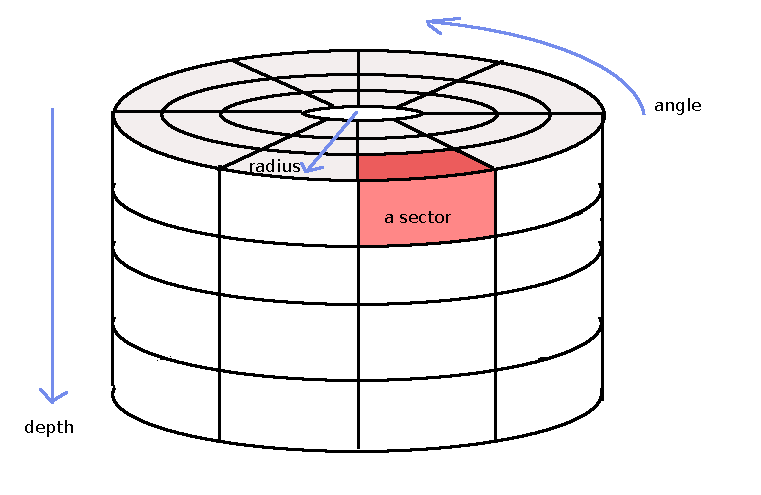=== Sets of sectors ===

If we specify a depth, radius and angle we uniquely identify exactly one sector. However, what happens if we don't specify all three? Then we identify a collection of the sectors on the disk. If we think about the sectors on the disk as forming a mathematical set, then we can think of these underspecified sector collections as subsets of the set of sectors on the disk. Let us consider the geometric interpretation of each possibility.

Case 0 - nothing specified: this gives all the sectors on the disk.

Case 1 - depth specified: this gives a vertical slice of sectors. For example,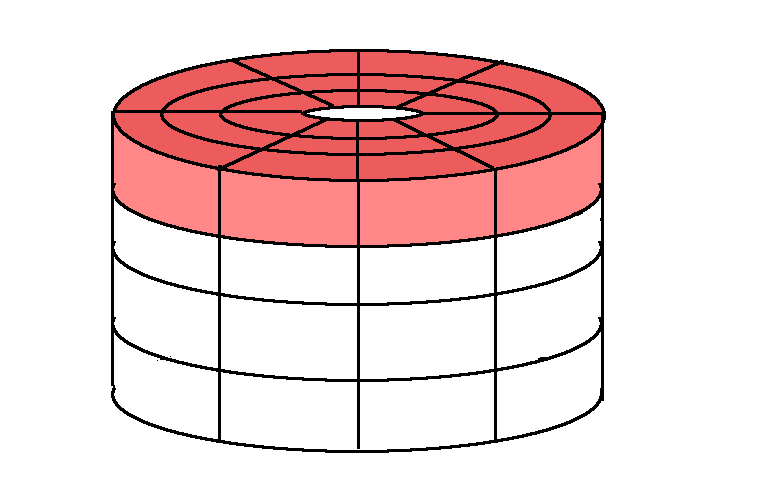Case 2 - radius specified: this gives a sub-cylinder of sectors. For example,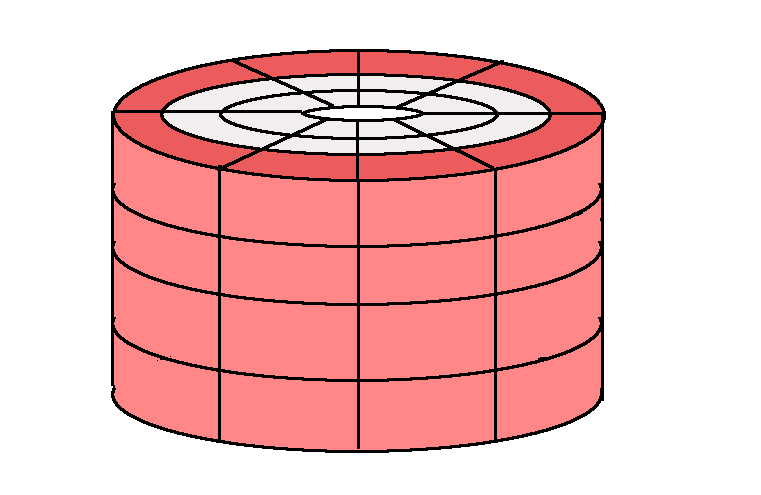Case 3 - angle specified: this gives a wedge of sectors. For example,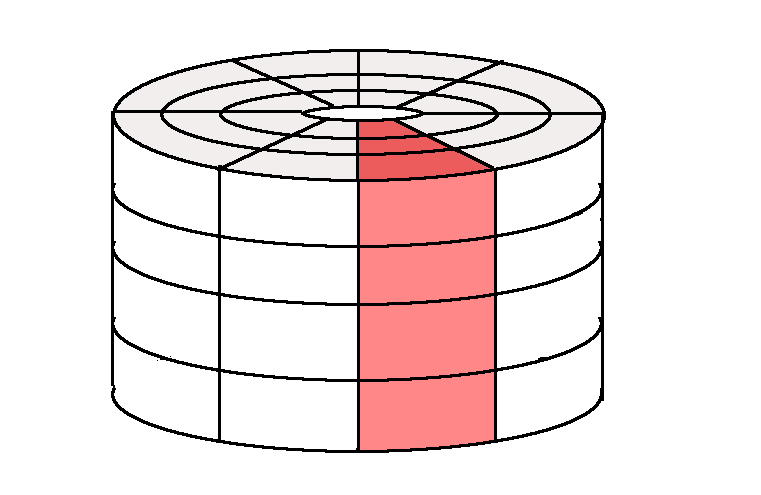Case 4 - depth and radius specified: this gives a vertical slice of a sub-cylinder of sectors. For example,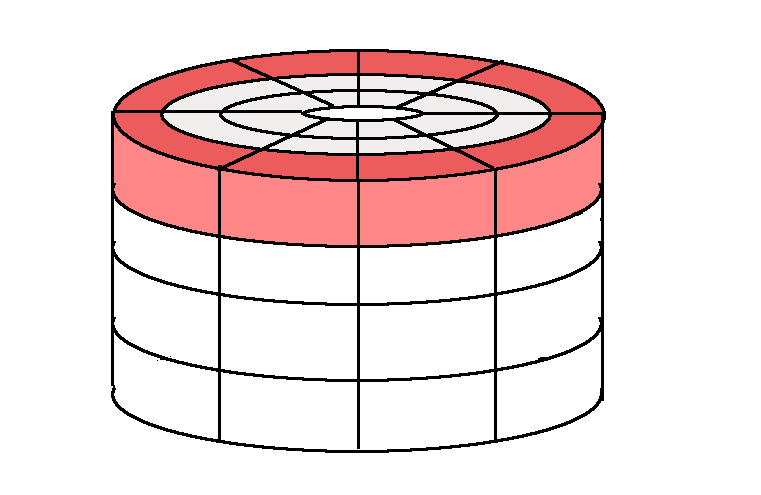Case 5 - radius and angle specified: this gives a vertical stack of sectors. For example,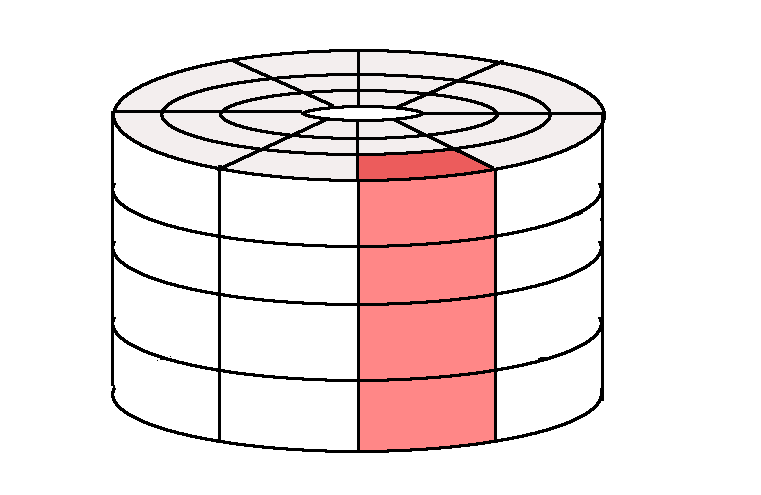Case 6 - angle and depth specified: this gives a vertical slice of a wedge of sectors. For example,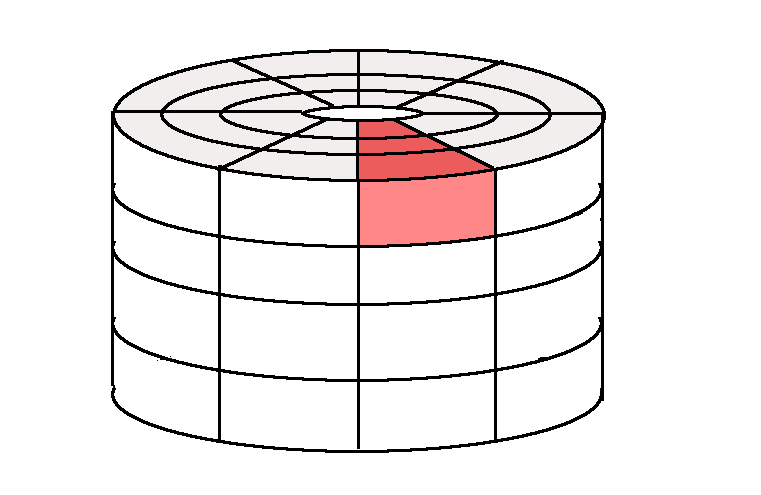Case 7 - depth, radius and angle all specified: this gives exactly one sector.

All this allows us to give a new interpretation of what a CHS address is actually doing. Instead of thinking of it as directly specifying a depth, radius and angle, we can think of as an intersection between 3 sets, one from Case 1, one from Case 2, and the last from Case 3.

For example, the Case 1 set (depth) is coloured in green, the Case 2 set (radius) is coloured in blue, and the Case 3 set (angle) is coloured in red.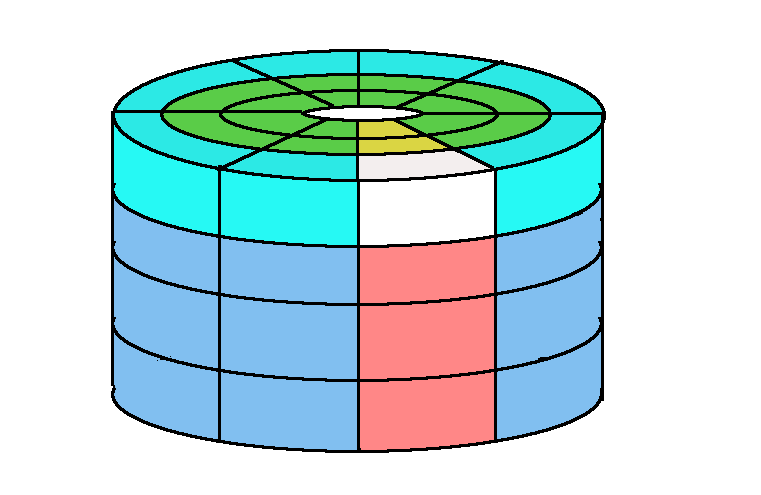=== The explanation ===

With this greater understanding under our metaphorical belt, we are ready to explain what IBM means by "cylinder", "head", "sector", "sectors per track" and "heads per cylinder".

First in a CHS address C, the "cylinder", is specified. This gives the radius at which the sector exists. We now have a Case 2 set of sectors.

Secondly, we specify H, the "head". This gives the depth at which the sector exists. But this is where the terminology becomes complicated. There are two things that we could mean by a "head" on a disk. We could either be referring to a Case 1 set where we have only specified the depth, OR we could be referring to a Case 4 set where both the radius and depth have been specified, since before selecting a "head" we must have already chosen a "cylinder". The name given to Case 4 set is a "track".

Finally, we specify S, the "sector". This gives the angle at which the sector exists. However, instead of thinking of "sector" as the angle itself, we are in Case 7 -- a unique sector -- because we have already specified the "cylinder" and "head" (altogether a "track"). Therefore, although the "sector" digit by itself gives a Case 3 set, along with its previous digits we do indeed specify a sector.

This naming is completely nonsensical. If S is called a "sector", then surely we should call H a "track", because with its prior digit we have identified a track! And going the other way, if H if called a "head", it seems as if we are calling each digit be the most general set it represents when standing alone, and therefore we should be calling S a "wedge" (or something along those lines).

We have further problems when we look into "SPT" and "HPC". "SPT" stands for "sectors per track", which actually makes a reasonable amount of sense. It does indeed tell you the number of sectors in each "track" -- a Case 4 set. However "HPC" stands for "heads per cylinder" which is an absurdity: it is asking for the number of heads (Case 1 sets) in each cylinder (Case 2 sets), and yet Case 1 sets are not subsets of Case 2 sets! A more reasonable name for "HPC" would be "tracks per cylinder", since it tells us the number of Case 4 sets in each Case 2 set. But it could also be called the number of "heads per disk", the number of distinct Case 1 sets, an equivalent definition.

*Yikes.*

=== Addendum A: Virtual geometry ===

Since solid state drives don't actually have any disk-like geometry, they don't have for values HPC and SPT. Unfortunately, because of Backwards Compatibility™, we need to emulate CHS addressing for things like SSDs and USB thumb drives. (In fact, we also have to emulate CHS addressing for optical media like CDs, because although they have disk-like structure, they weren't similar enough to old hard drives for IBM's liking.)

Sadly, we can't just make these drives report SPT to be larger than the total number of sectors, so that we can ignore C and H. No; that would be far to easy. We have the restriction that S must fit within 6 bits, and H within 8 bits. Therefore, HPC can be at most 256, and SPT at most 63. Wait, why can't SPT be 64? Recall, because of S starting at 1, the maximum value of S *is* SPT. Thus, SPT itself must be less than 2^6. So it's at most 63. When selecting virtual geometry for a drive, it is customary to select SPT and HPC to be their maximum values. Then, C will range from 0 up to X / 16128 - 1, where X is the total number of sectors on the drive.

=== Addendum B: Packed like sardines ===

As stated in Addendum A, S is a 6 bit value and H is an 8 bit value. Additionally, C is a 10 bit value. (This limits us to at most 63 * 256 * 1024 = 16515072 sectors (just less than 8GB) that can be addressed using CHS addressing.) This means that we can fit a CHS into a 3 byte value. You might think that the standard encoding of CHS would be something like (C << 14) | (H << 6) | (S << 0). But of course, IBM would not want it to be this simple. Instead, it's ((C & 0xFF) << 16) | (((C & 0x300) >> 8) << 14) | (S << 8) | (H << 0). (Admittedly, this encoding might have made it slightly easier to do on 16-bit processors, but it still annoys me.)